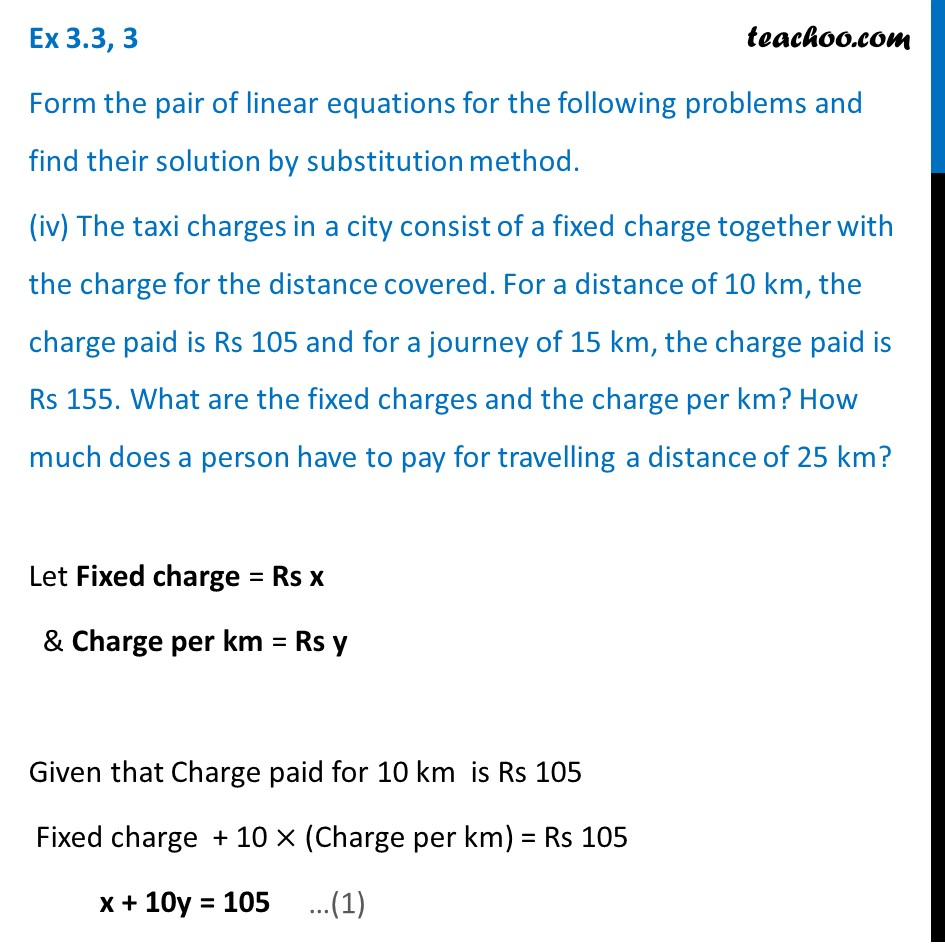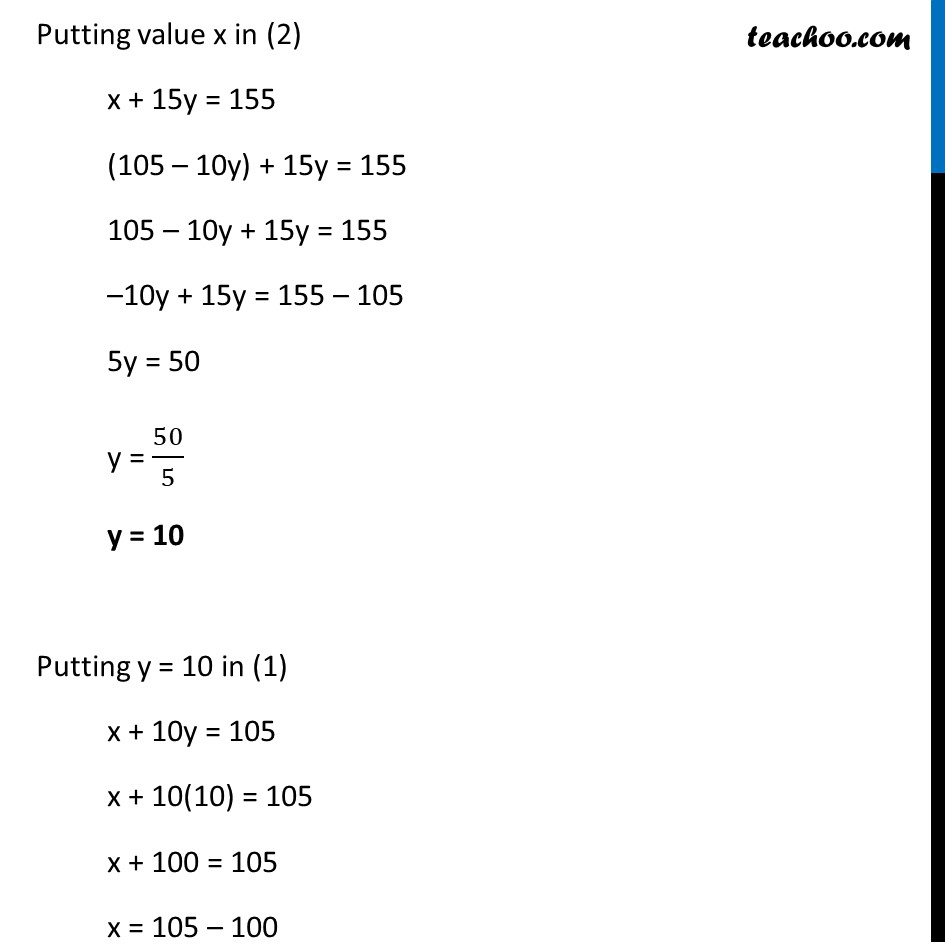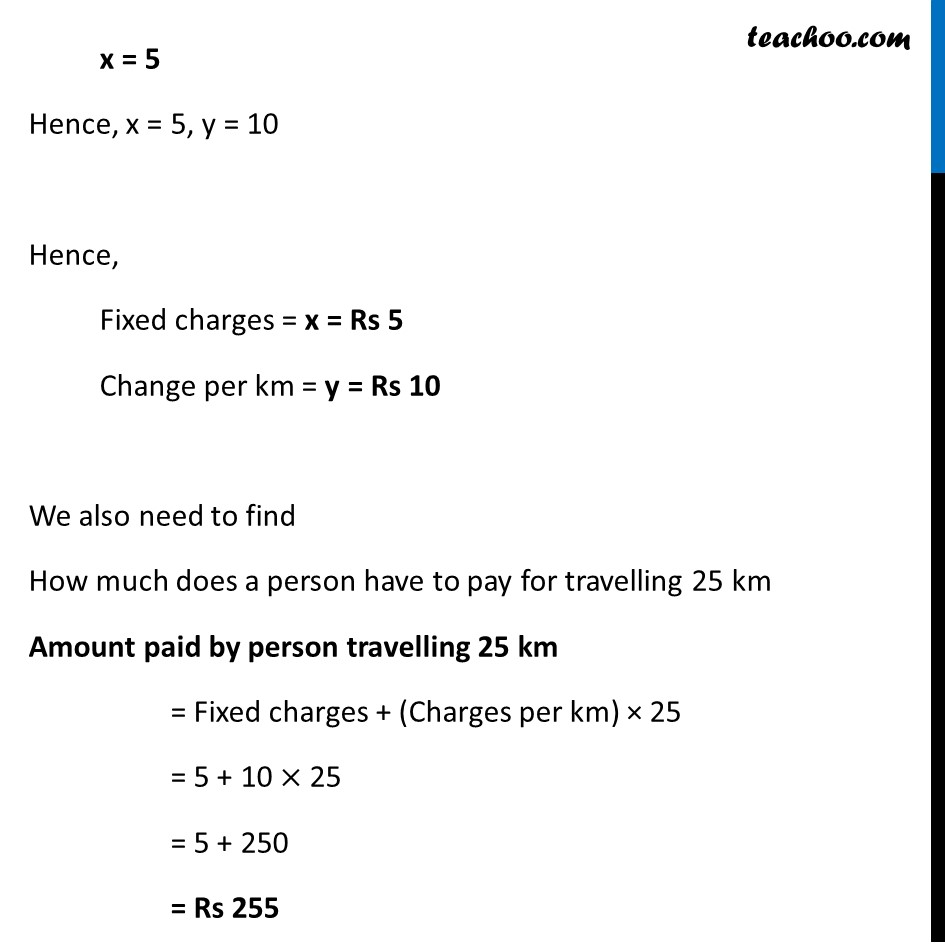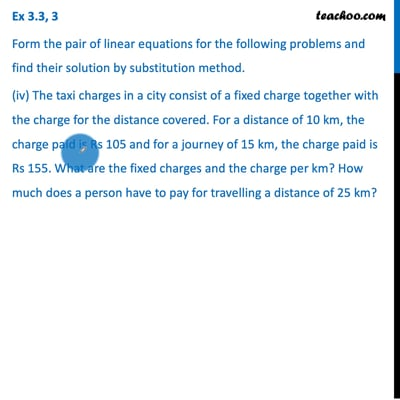Ex 3.3

Chapter 3 Class 10 Pair of Linear Equations in Two Variables
Serial order wiseThis video is only available for Teachoo black users

Get live Maths 1-on-1 Classs - Class 6 to 12

### Transcript

Ex 3.3, 3 Form the pair of linear equations for the following problems and find their solution by substitution method. (iv) The taxi charges in a city consist of a fixed charge together with the charge for the distance covered. For a distance of 10 km, the charge paid is Rs 105 and for a journey of 15 km, the charge paid is Rs 155. What are the fixed charges and the charge per km? How much does a person have to pay for travelling a distance of 25 km? Let Fixed charge = Rs x & Charge per km = Rs y Given that Charge paid for 10 km is Rs 105 Fixed charge + 10 × (Charge per km) = Rs 105 x + 10y = 105 Also, Given that Charge paid for 15 km is ₹ 155 Fixed charge + 15 × (Charge per km) = ₹ 155 x + 15y = 155 Our equations are x + 10y = 105 …(1) x + 15y = 155 …(2) From (1) x + 10y = 105 x = 105 – 10y Putting value x in (2) x + 15y = 155 (105 – 10y) + 15y = 155 105 – 10y + 15y = 155 –10y + 15y = 155 – 105 5y = 50 y = 50/5 y = 10 Putting y = 10 in (1) x + 10y = 105 x + 10(10) = 105 x + 100 = 105 x = 105 – 100 x = 5 Hence, x = 5, y = 10 Hence, Fixed charges = x = Rs 5 Change per km = y = Rs 10 We also need to find How much does a person have to pay for travelling 25 km Amount paid by person travelling 25 km = Fixed charges + (Charges per km) × 25 = 5 + 10 × 25 = 5 + 250 = Rs 255